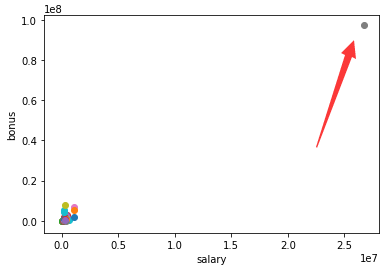# 机器学习安然数据集分析报告

## 数据集初步探索

### 加载数据集

with open("final_project_dataset.pkl", "r") as data_file:
data_dict = pickle.load(data_file)


### 数据集初步分析

{METTS MARK：{'salary': 365788, 'to_messages': 807, 'deferral_payments': 'NaN', 'total_payments': 1061827, 'exercised_stock_options': 'NaN', 'bonus': 600000, 'restricted_stock': 585062, 'shared_receipt_with_poi': 702, 'restricted_stock_deferred': 'NaN', 'total_stock_value': 585062, 'expenses': 94299, 'loan_advances': 'NaN', 'from_messages': 29, 'other': 1740, 'from_this_person_to_poi': 1, 'poi': False, 'director_fees': 'NaN', 'deferred_income': 'NaN', 'long_term_incentive': 'NaN', 'email_address': 'mark.metts@enron.com', 'from_poi_to_this_person': 38}}


POI 标签 : [‘poi’] (boolean，整数)

### POI统计

POI( Person of interest )是嫌疑犯的意思，数据集有一个标签（label）就是嫌疑犯，所以我们只需要统计
data_dict[preson name][‘poi’] == 1

## 异常值调查和处理data_dict = sorted(data_dict.items(), key = lambda x : x["salary"] ,reverse=True)

for x in data_dict :
if x['salary'] > 1000000 and x['bonus'] > 5000000 :
print x, x['salary'], x['bonus']


data_dict = dict(data_dict)

data_dict.pop('TOTAL', '52')



## 优化特征选择

### 创建新的特征

def poi_email_ratio(from_poi_to_this_person, to_messages):
if from_poi_to_this_person or to_messages == 'NaN':
to_poi_ratio = 0
else:
to_poi_ratio = float(from_poi_to_this_person)/to_messages
return to_poi_ratio

# create new key and value
for key in my_dataset:
my_dataset[key]['to_poi_ratio'] = poi_email_ratio(my_dataset[key]['from_poi_to_this_person'],  my_dataset[key]['to_messages'])



### 添加新特征之后的数据集
data = featureFormat(my_dataset, features_list, sort_keys = True)
labels, features = targetFeatureSplit(data)

### 未添加新特征的数据集
data = featureFormat(data_dict, features_list, sort_keys = True)
labels, features = targetFeatureSplit(data)


### new feature
The naive_bayes's recall is: 0.871794871795
The naive_bayes's precision is : 0.871794871795
The Decession_tree's recall is: 0.897435897436
The Decession_tree's precision is : 0.897435897436

### orignal feature
The naive_bayes's recall is: 0.871794871795
The naive_bayes's precision is : 0.871794871795
The Decession_tree's recall is: 0.846153846154
The Decession_tree's precision is : 0.846153846154


## 选择和调整算法

### 选择算法

from sklearn.naive_bayes import GaussianNB
clf = GaussianNB()

clf.fit(features_train, labels_train)
y_pred = clf.predict(features_test)
recall = recall_score(labels_test, y_pred, average='micro')
precision = precision_score(labels_test, y_pred, average='micro')
print "The naive_bayes's recall is: %s " % recall
print "The naive_bayes's precision is : %s" % precision


from sklearn import tree
from sklearn.model_selection import GridSearchCV
trees = tree.DecisionTreeClassifier()
parameters = {'min_samples_split' : range(5,80,5), 'splitter' : ('best', 'random')}

clf = GridSearchCV(trees, parameters)
clf.fit(features_train, labels_train)
y_pred = clf.predict(features_test)
recall = recall_score(labels_test, y_pred, average='micro')
precision = precision_score(labels_test, y_pred, average='micro')
print "The Decession_tree's recall is: %s " % recall
print "The Decession_tree's precision is : %s" % precision


### 使用 GridSearchCV 进行参数调整

GridSearchCV 用于系统地遍历多种参数组合，通过交叉验证确定最佳效果参数。它的好处是，只需增加几行代码，就能遍历多种组合。当然与此对应的是机器学习过程所消耗的时间会相对较多。下面我们用GridSearchCV对决策树参数进行调整：

from sklearn import tree
from sklearn.model_selection import GridSearchCV
trees = tree.DecisionTreeClassifier()
parameters = {'min_samples_split' : range(5,80,5), 'splitter' : ('best', 'random')}

clf = GridSearchCV(trees, parameters)
clf.fit(features_train, labels_train)
print clf.score(features_test, labels_test)


## 验证和评估

### 训练集和数据集的拆分

from sklearn.model_selection import train_test_split
features_train, features_test, labels_train, labels_test = train_test_split(
features, labels, test_size=0.3, random_state=42)## 参考资料

Recall和Precision的理解 http://blog.csdn.net/Relocy/article/details/51453950

Precision-Recall metric: http://scikit-learn.org/stable/auto_examples/model_selection/plot_precision_recall.html